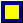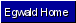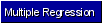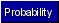Egwald Statistics: Probability and Stochastic Processes by Elmer G. Wiens Egwald's popular web pages are provided without cost to users. Please show your support by joining Egwald Web Services as a Facebook Fan:Follow Elmer Wiens on Twitter:Sampling Distributions. A. Chi-Square Distribution The chi-square distribution is the gamma distribution with alfa = .5 and beta = nu / 2, where nu = degrees of freedom. If the random variable X has the N(0, 1) distribution, then X^2 has the chi-square distribution with nu = 1 degrees of freedom. If each random variable of { X1, ..., Xn} has the N(0,1) distribution, and Y = X1^2 + . . . + Xn^2 then the random variable Y has the chi-square distribution with nu = n degrees of freedom. The density of the chi-square distribution is: f(y) = K * .5^nu/2 * y^(nu-2)/2 * exp(-y/2) where K = 1 / G(nu/2) and G = the gamma function. Density of the Chi-Square Distribution with nu degrees of freedom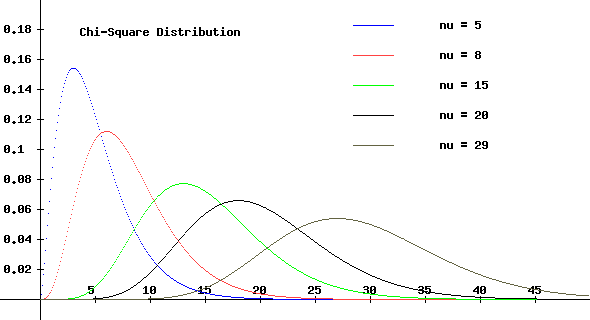B. Student-t Distribution The density of the Student-t distribution is: f(t) = G(nu/2 + 1/2) * (1 + t^2 / nu)^-(nu+1)/2 / sqrt(nu * pi) * G(nu/2) where G is the gamma function and nu = degrees of freedom. Density of the Student-t Distribution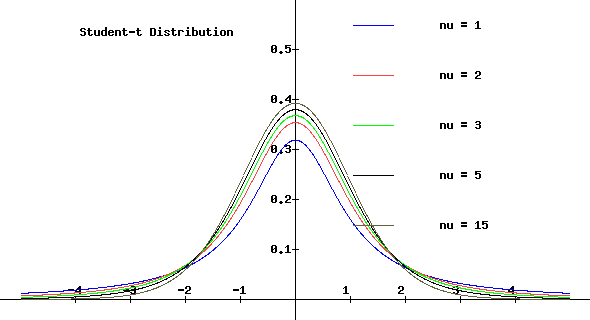If the random variable X has the standard normal distribution, and the random variable Y has the chi-square distribution with nu degrees of freedom, then the random variable T defined as: T = X / sqrt(Y/nu) has the Student-t distribution with nu degrees of freedom. C. F Distribution The density of the F distribution with r and s degrees of freedom is: f(x) = c * x^(r/s)-1 / (1 + r * x / s)^(r + s)/2 where c = G((r + s)/2) * (r/s)^(r/2) / [G(r/2) * G(s/2)] and G is the gamma function. Density of the F Distribution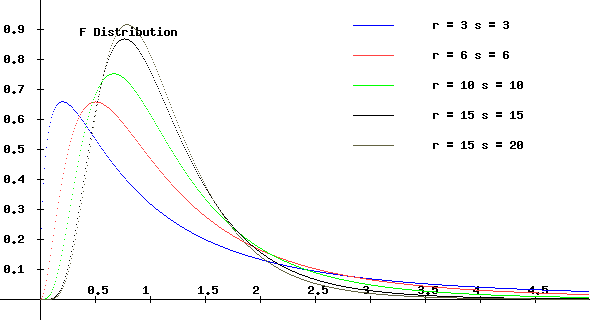If the independent random variables U and V have chi-square distributions with r and s degrees of freedom, respectively, then the random variable F = (U / r) / (V / s) has the F distribution with r and s degrees of freedom.Copyright © Elmer G. Wiens:   Egwald Web ServicesAll Rights Reserved.    Inquiries